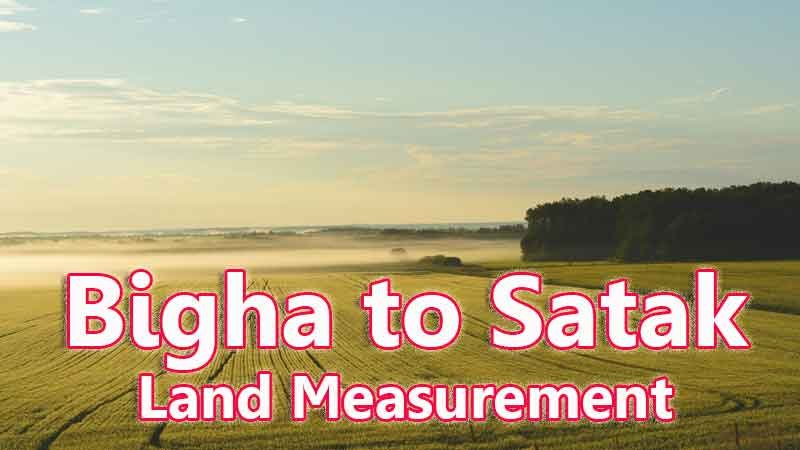# Bigha To Satak Converter

Bigha is a traditional land unit used to measure the land Area standardly. Here we are using the Bigha to Satak unit value conversion. And Bigha also comes under these land area units like Gonda, Kani, and Satak. In West Bengal, One Bigha is directly equivalent to Thirty Three Decimal Point Six Satak.

1 Bigha symbol in West Bengal is equal to 14400 square feet and it is widely used for land measurement units in West Bengal specifically. And these Satak, Bigha, Gonda, and more units are routinely used in the Indian states of West Bengal and Bangladesh for land measurement.

Input Here

bigha

Output

satak

1 Bigha = 33.05785123966942 Satak
Rounded Value Is (1 bigha = 33 satak)

## Measurement

Bigha means - a unit of land measurement. This tool provides great calculation over bigha to satak, 1 bigha to satak, 1 bigha in satak, 1 bigha equal to and etc. To convert bigha to satak, then multiply the unit value by 33.05785123966942. Then, 1 bigha * 33.05785123966942 = 33.05785123966942 Satak. Hence, 1 bigha is equal to 33.05785123966942 Satak.

Satak also comes under this land measurement unit and we are comparing values between bigha traditional unit with other side land measurement unit. By your wish, you can calculate the other two land units that are also possible under this page.

## Formula For Bigha to Satak Conversion(bigha to satak formula)

The base formula for this bigha to satak converter is

Satak = Bigha * 33.05785123966942

## How many satak in a bigha?

Step 1: To Convert 1 bigha to satak

Step 2: Applying formula satak = bigha * 33.05785123966942, (i.e) multiply the unit value by 33.05785123966942.

Step 3: Then, satak = 1 * 33.05785123966942 = 33.05785123966942.

Step 4: Hence, 1 bigha is equal to 33.05785123966942 satak.Some bigha to satak Conversion Chart for your reference:

 1 bigha = 33.05785123966942 satak 2 bigha = 66.11570247933884 satak 3 bigha = 99.17355371900825 satak 4 bigha = 132.23140495867767 satak 5 bigha = 165.2892561983471 satak 6 bigha = 198.3471074380165 satak 7 bigha = 231.40495867768593 satak 8 bigha = 264.46280991735534 satak 9 bigha = 297.5206611570248 satak 10 bigha = 330.5785123966942 satak

The bigha to satak conversion chart is above listed for your reference. This chart, however, represents the simple math calculation involved in the bigha to satak convertion online.

For Example: How much is 1 bigha to satak

Solution:

= (bigha * 33.05785123966942)

= (1 x 33.05785123966942)

= 33.05785123966942 Satak

For Example: How much is 120 bigha in satak

Solution:

= (bigha * 33.05785123966942)

= (120 x 33.05785123966942)

= 3966.9421487603304 satak

This formula provides an instant answer for all your questions / People also search:

• 1 bigha to satak
• 1 bigha is equal to how many satak
• Formula to convert bigha to satak online
• How to convert bigha to satak?
• How much satak is equal to a one bigha?
• How many satak in bigha?
• 1 bigha equal to satak